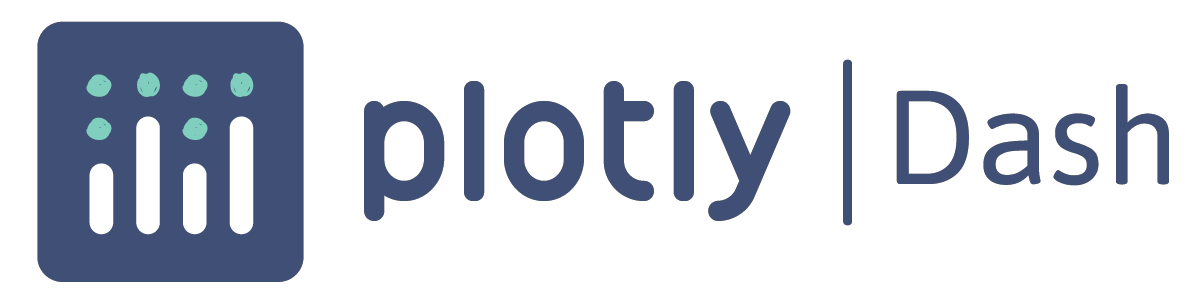# Create a Dashboard with Dash

Use Dash to create dashboards in Python and deploy them on Saturn CloudTry this example in seconds on Saturn Cloud

## Overview

With Dash from Plotly, data scientists can produce low-code data apps by abstracting away much of the technologies and protocols typically required for interactive data visualization. Deploying a Dash app on Saturn Cloud provides not only a scalable backend for your app but also a url link for dissemination.

In this example, we create a simple UI showing a Uniform Manifold Approximation and Projection (UMAP) model projection of the famous MNIST digits and fashion datasets. The app will read the data, train the UMAP model, and produce a 3D graph of the result using plotly.

## Creating the App

All the app code is contained in a file called “app.py.” To deploy this app on Saturn Cloud, call `python app.py` as the command in a Saturn Cloud deployment. See Saturn Cloud docs for more detailed instructions on deploying this and other dashboards.

The “app.py” file contains several sections to create the Dash app.

### Import the Libraries

This exercise uses Dash, plotly, and UMAP to create a dashboard app.

``````import numpy as np
import pandas as pd
import plotly.express as px
from dash import Dash, Input, Output, dcc, html
from umap import UMAP
``````

### Define the App and Layout

Next, define the app, then specify the layout. Some of this code might seem familiar if you work with HTML. Dash uses functions like `html.div` to define html components.

You can use Dash Core Components (`dcc`) such as `Dropdown` and `Graph` to define user input and output components.

This example creates two columns. The first contains a dropdown for the user to select a dataset, and the second a visualization of the UMAP projection.

``````app = Dash(__name__)
app.title = "UMAP Projections"

app.layout = html.Div(
[
html.Div(
children=[
html.H1(
"UMAP Projections for MNIST and Fashion-MNIST Datasets",
style={"text-align": "center"},
),
dcc.Markdown(
"""
Uniform Manifold Approximation and Projection (UMAP) is a general-purpose dimension reduction algorithm. Similar to t-distributed stochastic neighbor embedding (t-SNE), you can use UMAP to visualize the relationships between data points. In this example, we are training a three-component UMAP model on MNIST datasets and then displaying the 3D graph of the result. The color of the point in the graph is based on the label. In the resulting graph, blobs of colors show that UMAP clustered data points with similar labels together.
""",
),
],
),
html.Div(
[
html.Div(
children=[
html.H1("Input"),
html.Label("Dataset"),
dcc.Dropdown(
["MNIST-Digits", "MNIST-Fashion"], "MNIST-Digits", id="dataset_dropdown"
),
],
),
html.Div(
children=[
html.H1("Output"),
children=[dcc.Graph(id="graph")],
type="circle",
),
],
),
],
style={"display": "flex", "flex-direction": "row"},
),
]
)
``````

### Define the Callbacks

Dash uses callbacks to run functions based on user input. For this example, we define a single callback to update the graph when the dataset changes.

Dash uses `Input` and `Output` to define callback inputs and outputs. The first parameter is the id of the associated component in the layout, and the second is the type of information passed to and from the function.

For this example, the callback downloads the correct dataset based on the dropdown selection, runs `fit_transform` on the dataset to create the projection, and finally creates a 3D scatter plot of the result.

``````@app.callback(
Output("graph", "figure"),
Input("dataset_dropdown", "value"),
)
def update_figure(selected_dataset):
if selected_dataset == "MNIST-Digits":
"https://saturn-public-data.s3.us-east-2.amazonaws.com/MNIST-1000/mnist-1000-input.csv"
)
"https://saturn-public-data.s3.us-east-2.amazonaws.com/MNIST-1000/mnist-1000-labels.csv"
)
y = np.unique(y, return_inverse=True)

elif selected_dataset == "MNIST-Fashion":
"https://saturn-public-data.s3.us-east-2.amazonaws.com/MNIST-1000/fashion-1000-input.csv"
)
"https://saturn-public-data.s3.us-east-2.amazonaws.com/MNIST-1000/fashion-1000-labels.csv"
)
y = np.unique(y, return_inverse=True)

else:
return None, "Please select a dataset."

umap_3d = UMAP(n_components=3, init="random", random_state=0)

proj_3d = umap_3d.fit_transform(X, y=y)

fig = px.scatter_3d(proj_3d, x=0, y=1, z=2, color=y)

fig.update_layout(transition_duration=500, height=1000)
fig.update(layout_coloraxis_showscale=False)
fig.update_traces(marker_size=2)

return fig
``````

### Run the App

Finally, define the code to run the app. The important points here are the host and port numbers. For users to access the server, the host value must be `"0.0.0.0"` and the port number `"8000"`. `8000` is the only port exposed on Saturn Cloud deployments.

``````if __name__ == "__main__":
app.run_server(host="0.0.0.0", port="8000")
``````

Then load the app code and data to git, and link the code appropriately to a Saturn Cloud deployment.

Check out our other dashboard resources for examples using other frameworks.

``````import numpy as np
import pandas as pd
import plotly.express as px
from dash import Dash, Input, Output, dcc, html
from umap import UMAP

app = Dash(__name__)
app.title = "UMAP Projections"

app.layout = html.Div(
[
html.Div(
children=[
html.H1(
"UMAP Projections for MNIST and Fashion-MNIST Datasets",
style={"text-align": "center"},
),
dcc.Markdown(
"""
Uniform Manifold Approximation and Projection (UMAP) is a general-purpose dimension reduction algorithm. Similar to t-distributed stochastic neighbor embedding (t-SNE), you can use UMAP to visualize the relationships between data points. In this example, we are training a three-component UMAP model on MNIST datasets and then displaying the 3D graph of the result. The color of the point in the graph is based on the label. In the resulting graph, blobs of colors show that UMAP clustered data points with similar labels together.
""",
),
],
),
html.Div(
[
html.Div(
children=[
html.H1("Input"),
html.Label("Dataset"),
dcc.Dropdown(
["MNIST-Digits", "MNIST-Fashion"], "MNIST-Digits", id="dataset_dropdown"
),
],
),
html.Div(
children=[
html.H1("Output"),
children=[dcc.Graph(id="graph")],
type="circle",
),
],
),
],
style={"display": "flex", "flex-direction": "row"},
),
]
)

@app.callback(
Output("graph", "figure"),
Input("dataset_dropdown", "value"),
)
def update_figure(selected_dataset):
if selected_dataset == "MNIST-Digits":
"https://saturn-public-data.s3.us-east-2.amazonaws.com/MNIST-1000/mnist-1000-input.csv"
)
"https://saturn-public-data.s3.us-east-2.amazonaws.com/MNIST-1000/mnist-1000-labels.csv"
)
y = np.unique(y, return_inverse=True)

elif selected_dataset == "MNIST-Fashion":
"https://saturn-public-data.s3.us-east-2.amazonaws.com/MNIST-1000/fashion-1000-input.csv"
)
"https://saturn-public-data.s3.us-east-2.amazonaws.com/MNIST-1000/fashion-1000-labels.csv"
)
y = np.unique(y, return_inverse=True)

else:
return None, "Please select a dataset."

umap_3d = UMAP(n_components=3, init="random", random_state=0)

proj_3d = umap_3d.fit_transform(X, y=y)

fig = px.scatter_3d(proj_3d, x=0, y=1, z=2, color=y)

fig.update_layout(transition_duration=500, height=1000)
fig.update(layout_coloraxis_showscale=False)
fig.update_traces(marker_size=2)

return fig

if __name__ == "__main__":
app.run_server(host="0.0.0.0", port="8000")

``````